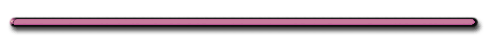HomeRankingTask

Rank the total resistance  from smallest to largest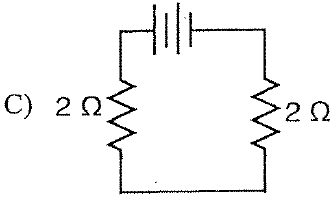Name this Circuit

School blocks YouTube? Use file below.

Show Me The Physics YouTube Channel

Ex 6)  Series Circuit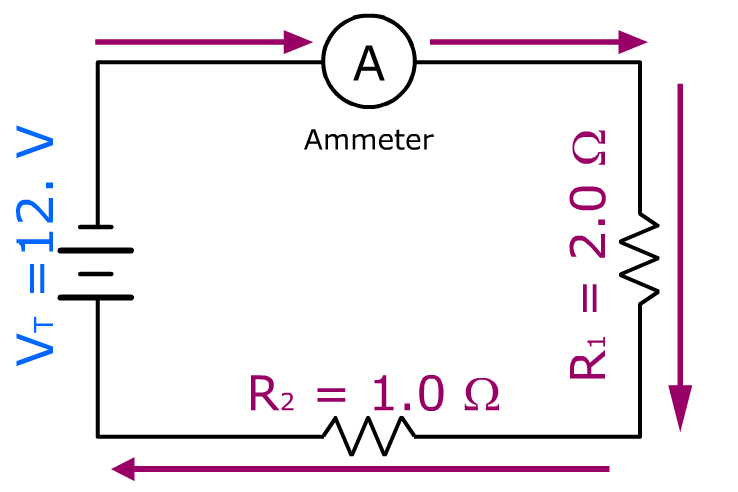a) Find the 'equivalent resistance'

RT = R1 + R2

= 2.0 Ω + 1.0 Ω

= 3.0 ohms

b) Find the current (IT) going through this circuit

VT = ITRT

12. Volt = IT(3.0 ohms)

IT = 4.0 amps

c) Find potential drop across R1 & R2

V = IR

V1=4.0 amps(2.0 ohms)

= 8.0 Volts

V2 =4.0 amps(1.0 ohms)

= 4.0 Volts

Ex 7)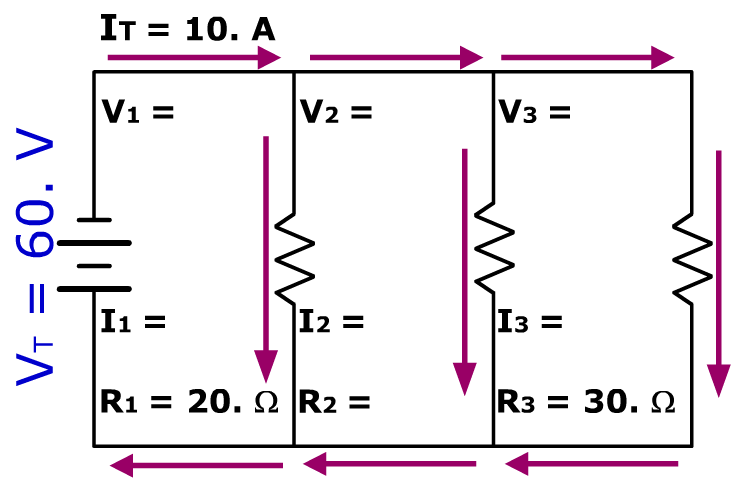a) Find combined resistance (RT)

VT = IT RT

60. volts = (10 amps)RT

= 6.0 ohms

b) Find the current in R1

V1 = I1R1

60. Volts = I120. ohms

I1 = 3.0 amps

c) Find I3

V3 = VT = 60. volts

Use Ohm's Law

V3 = I3R3

60. volts = I330. ohms

I3  = 2.0 amps

d) Find R2

e) Find value of the second resistor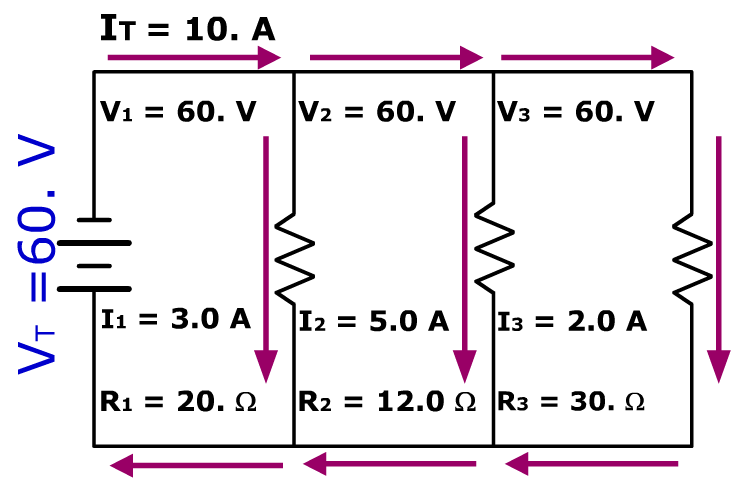In a Series Circuit: (or anytime current in resistors are equal) V = I R   Potential difference (V)  across a resistor  is proportional to resistance   In parallel, V are always equal

Ex 8)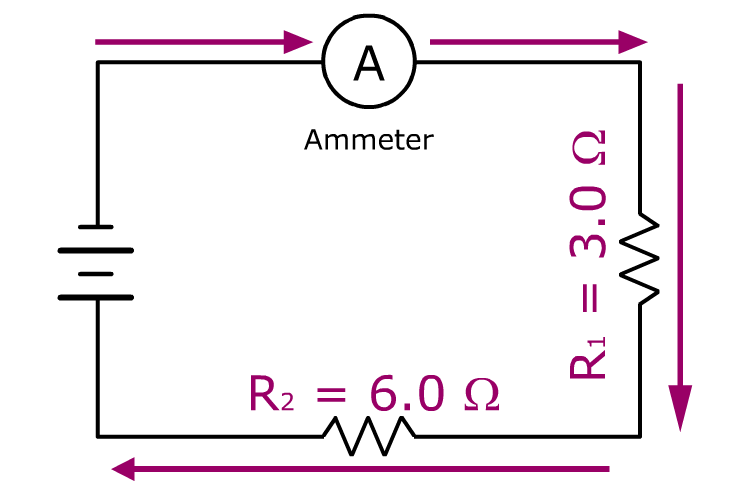If the voltage drop across the 3 ohms resistor is 4.0 volts then the voltage drop across the 6.0 ohm resistor is

3.0 ohms : 4.0 volts

6.0 ohms : x

x = 8.0 volts

a) Find the total voltage in this series circuit

VT = V1 + V2

VT = 8.0 V + 4.0 V

= 12 V

b) Find combined resistance in this circuit

RT = 6.0 ohms + 3.0 ohms

RT = 9.0 ohms

c) Total current in this circuit?

VT = ITRT

12 V = IT(9.0 ohms)

I= 1.3 Amps

Ex 9)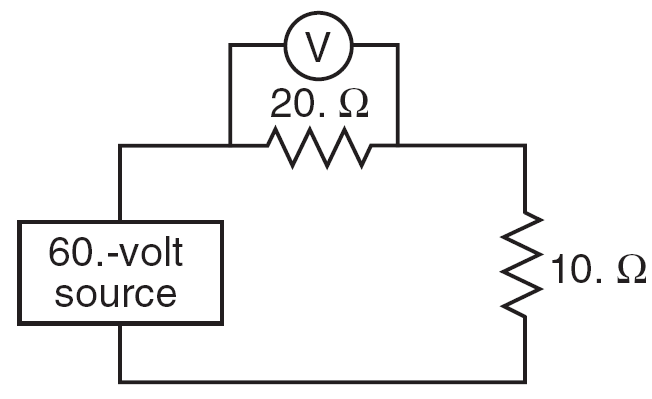a) Compare the current through each resistor

Same (Series Circuit)

IT = I1 = I2 = ...

b) Compare the voltage drops in this circuit?20. ohm resistor has twice the potential difference (voltage)

c) Current in this circuit?VT = ITRT

60. V =  IT(30. ohms)

IT  = 2.0 Amps

c) Potential difference in 20. ohm resistor?V = IR

= 2.0 amps(20. ohms)

= 40. V

Ex 10) Find R2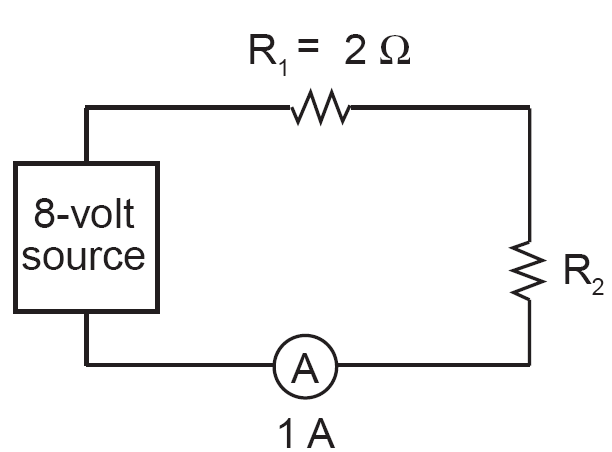V = IR

First Find RT

8 V = 1 Amp(RT)

R= 8 ohms

Find R2RT = R1 + R2

Since

R= 8 ohms

R = 6 ohms

AP Circuits

EMF - max. voltage of source

- internal resistance causes actual V to be lower

Complex Circuits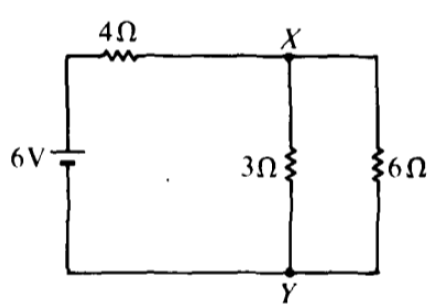Ex) Find the potential difference between  X and Y

Convert parallel R to a single R

1/RT = 1/3 + 1/6

1/RT = 3/6

RT = 2 ohmsSeries Circuit 4 ohms,
2 ohms, 6 V source

Need V across 2 ohm R

V  = IR

VT  = ITRT

6 V  = IT6 ohms

IT = 1 ampV = 2 V

Ex 2)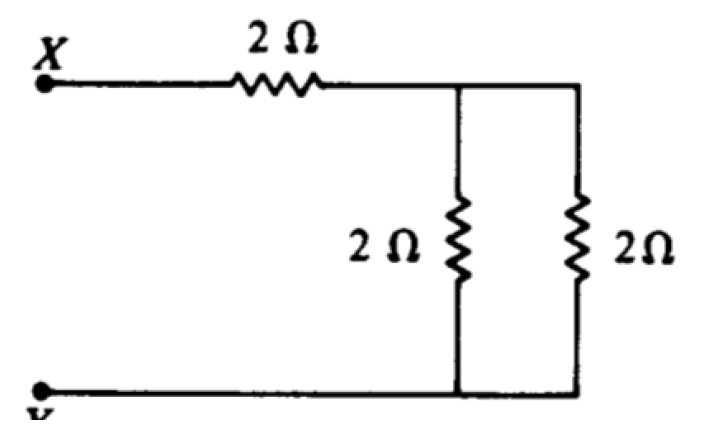Find equivalent R between X and Y

1/RT = 1/3 + 1/6

RT = 2 ohms

RT = 3 ohms

Ex 3)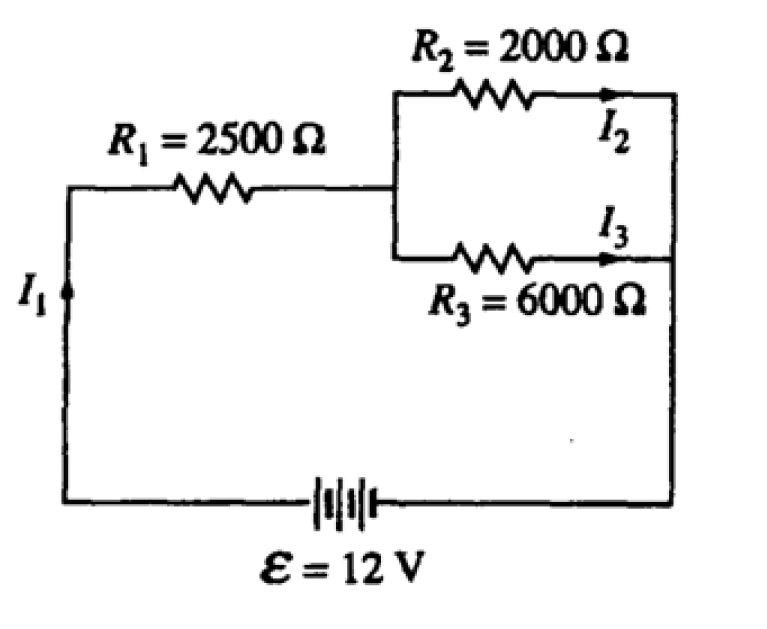Find total current

(A)  0.8 mA   (B)  1.0 mA

(C)  2.0 mA  (D) 3.0 mA (E) 6.0 mA

Reduce parallel R to single R

1/RT=1/2000+1/6000

1/RT=3/6000+1/6000

RT = 1500 ohmsV = IR

12 V = I[4000 ohms]

(A)  0.8 mA   (B)  1.0 mA

(C)  2.0 mA  (D) 3.0 mA (E) 6.0 mAAttaching MetersEnrichment